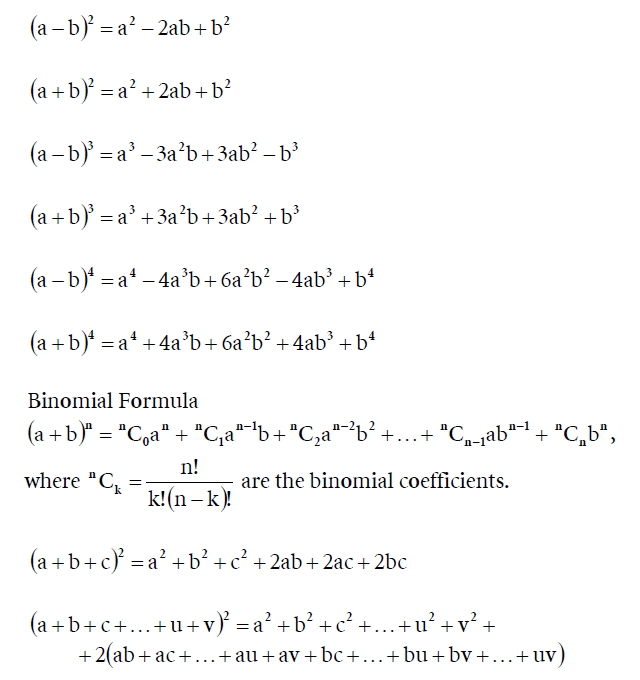## Formule de calcul prescurtat

Elemente de Matematică și Informatică. Teorie, Probleme. Discuții.
Posts: 750
Joined: 22 Jan 2012, 14:23
Location: România
Contact:

###Formule de calcul prescurtat

Posts: 750
Joined: 22 Jan 2012, 14:23
Location: România
Contact:

### Formule de calcul prescurtat

(Radicalul trebuia pus între paranteze pentru a fi ridicat la putere... Imaginea a fost preluată iniţial de pe un site...)

Posts: 750
Joined: 22 Jan 2012, 14:23
Location: România
Contact:

### Formule de calcul prescurtatPosts: 750
Joined: 22 Jan 2012, 14:23
Location: România
Contact:

### Formule de calcul prescurtatPrin definiție, n factorial este definit: n! = 1*2*3*...*n

Posts: 750
Joined: 22 Jan 2012, 14:23
Location: România
Contact:

### Formule de calcul prescurtatPosts: 750
Joined: 22 Jan 2012, 14:23
Location: România
Contact:

### Formule de calcul prescurtat

$(1 + a)(1+a^2+a^4) = 1 + a + a^2 + a^3 + a^4 + a^5$

Posts: 750
Joined: 22 Jan 2012, 14:23
Location: România
Contact:

### Formule de calcul prescurtat

$(primul + secundul)^2 = primul^2 + 2 \cdot primul \cdot secundul + secundul^2$

$(1 + 4x^3) = 1^2 + 2 \cdot 1 \cdot 4x^3 + (4x^3)^2 = 1 + 8x^3 + 16x^6$

Posts: 750
Joined: 22 Jan 2012, 14:23
Location: România
Contact:

### Formule de calcul prescurtat

Rezolvați în $\mathbb{R}$ ecuația $x^4 = 81$

$x^4 = 81 \Longleftrightarrow$

$x^4 - 81 =0 \Longleftrightarrow$

$(x^2)^2 - 9^2 = 0 \Longleftrightarrow$

$(x^2 - 9)(x^2+9) = 0 \Longleftrightarrow$

$(x^2 - 3^2)(x^2+9) = 0 \Longleftrightarrow$

$(x - 3)(x+3)(x^2+9) = 0$

și cum $x^2+9 > 0$ deci ultima paranteză nu poate fi nulă,

obținem unicele soluții reale $x=3$ respectiv $x=-3$

Posts: 750
Joined: 22 Jan 2012, 14:23
Location: România
Contact:

### Formule de calcul prescurtat

Admin » 18 Sep 2016, 23:14 wrote:Rezolvați ecuația $x^4 = 81$

$x^4 = 81 \Longleftrightarrow$
...

În mulțimea numerelor complexe $\mathbb{C}$ toate cele 4 soluții sunt: $3, -3, 3i, -3i$ (unde $i=\sqrt{-1}$)

Posts: 750
Joined: 22 Jan 2012, 14:23
Location: România
Contact:

### Formule de calcul prescurtat